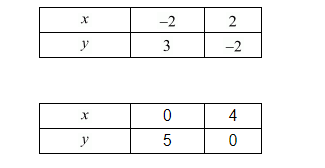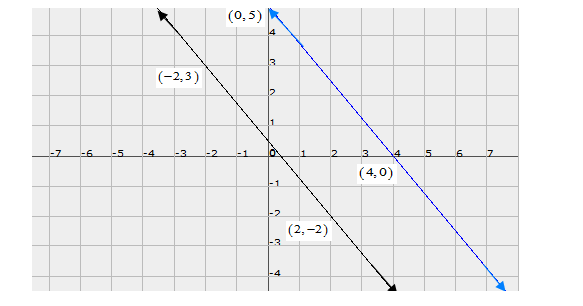# Gloria is walking along the path joining (−2, 3) and (2, −2),`
Question:

Gloria is walking along the path joining (−2, 3) and (2, −2), while Suresh is walking along the path joining (0, 5) and (4, 0). Represent this situation graphically.

Solution:

Gloria is walking the path joining $(-2,3)$ and $(2,-2)$

Suresh is walking the path joining $(0,5)$ and $(4,0)$The graphical representations are